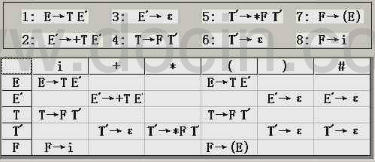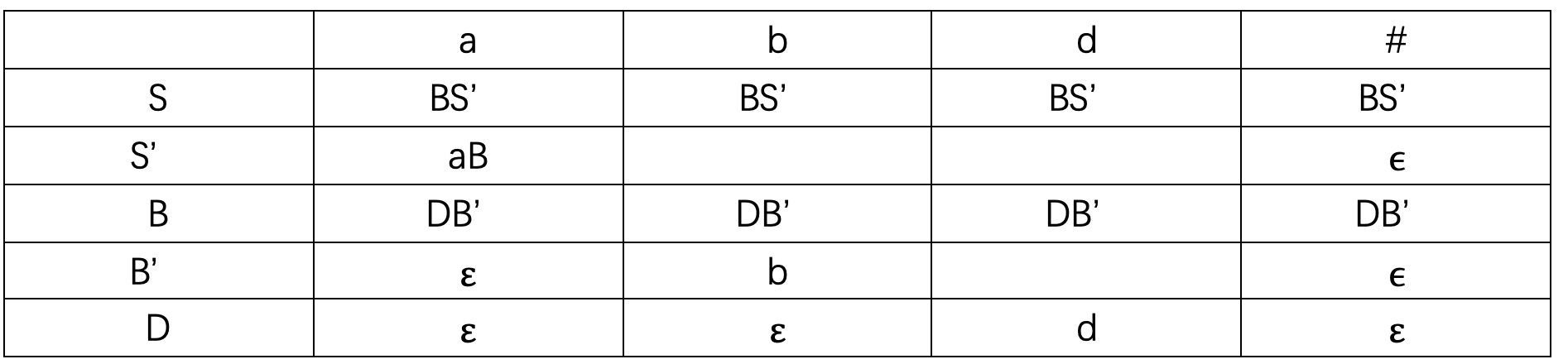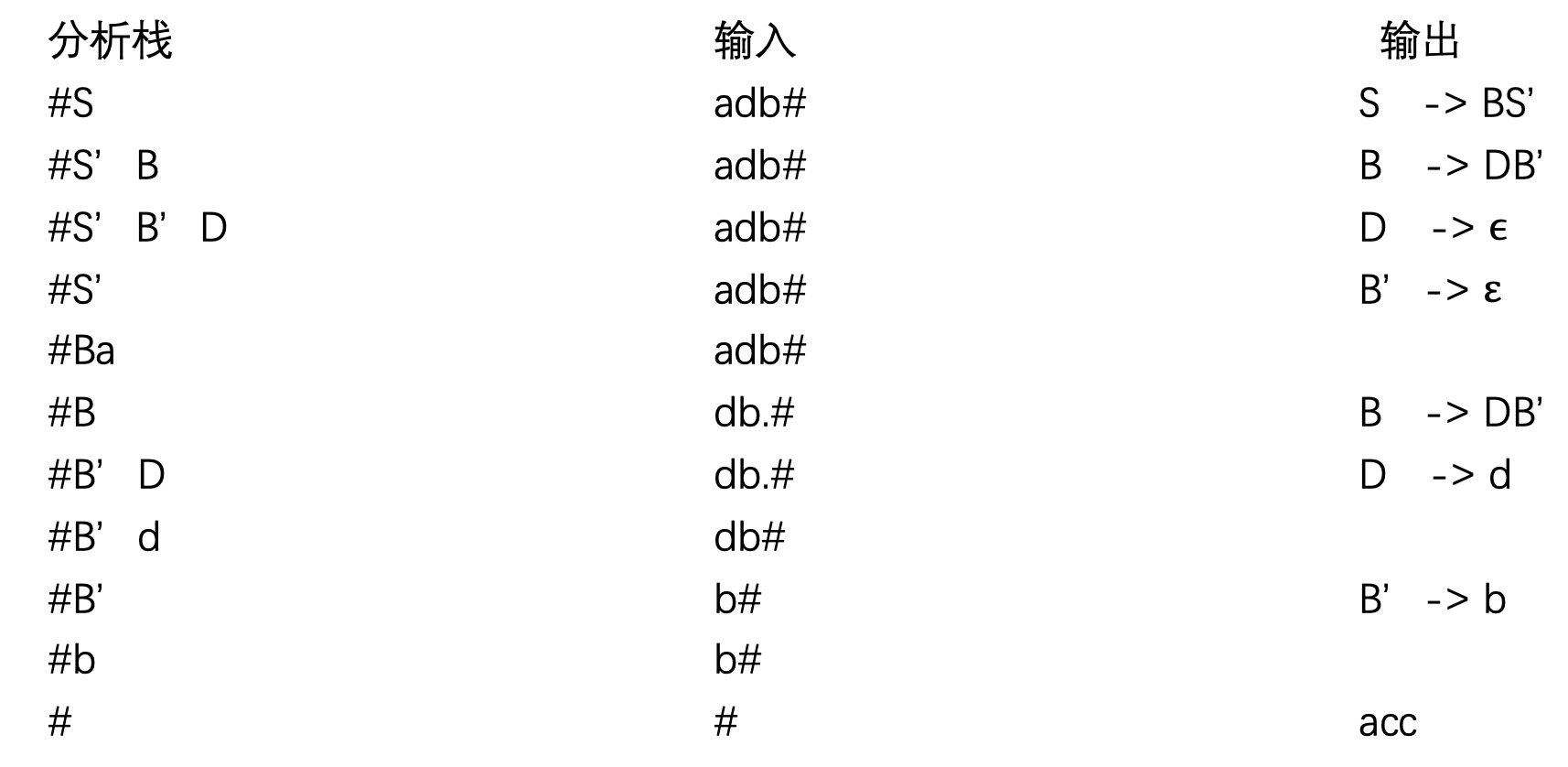• 编译原理FIRST集和FOLLOW集的求法
千次阅读 多人点赞
2019-06-18 17:16:41

# 编译原理FIRST集和FOLLOW集的求法

由于最近要期末考试了，所以要从头到尾复习（预习）一遍了。陈意云老师写的课本比较抽象，看不太懂，最后又找了中科大的编译原理课，可能听的时候太困了没有集中精力，又跑去看了以前曾经看过的哈工大的公开课，应该是看懂了，所以就厚颜无耻的来捋一捋，欢迎交流，批评指正。

废话不多说，直接看怎么计算的吧。
首先看FIRST集，文字描述如下：

1.FIRST(X)：可以从X推导出的所有串首终结符构成的集合
如果X=>*ε，那么ε∈FIRST(X)

举个栗子

表达式FIRST集FOLLOW集
E -> TE’{}{}
E’-> +TE’|ε{}{}
T-> FT’{}{}
T’->*FT’|ε{}{}
F-> (E)|id{}{}

我是这样做的，首先看有终结符的表达式F-> (E)|id可以得到FIRST(F)={(, id}；
再看有终结符的表达式T’->FT’|ε，得到FIRST(T’)={*, ε}
再看E’-> +TE’|ε，得到FIRST(E’)={+ , ε}，所得得到以下结果：

表达式FIRST集FOLLOW集
E -> TE’{}{}
E’-> +TE’|εFIRST(E’)={+ , ε}{}
T-> FT’{}{}
T’->*FT’|εFIRST(T’)={*, ε}{}
F-> (E)|idFIRST(F)={(, id}{}

然后再从头看每个表达式可以得到FIRST(E)=FIRST(T)，所以去求FIRST(T)；
所以来看表达式T-> FT’，得到FIRST(T)=FIRST(F)，又因为F无法推导出ε，所以FIRST(T)=FIRST(F)；
同理可得FIRST(E)=FIRST(T)=FIRST(F)
所以表就更新为以下结果：

表达式FIRST集FOLLOW集
E -> TE’FIRST(E)={(, id}{}
E’-> +TE’|εFIRST(E’)={+ , ε}{}
T-> FT’FIRST(T)={(, id}{}
T’->*FT’|εFIRST(T’)={*, ε}{}
F-> (E)|idFIRST(F)={(, id}{}

这时候，我们FIRST集就已经求完了，接下来看FOLLOW集：
同样先来文字描述：

FOLLOW(A)：可能在某个句型中紧跟在A后边的终结符a的集合
FOLLOW(A)={a| S=>*αAaβ, a∈VT, α,β∈(VT∪VN)*
如果A是某个句型的最右符号，则将结束符“$”添加到FOLLOW(A)中。 还是上面的栗子： 表达式FIRST集FOLLOW集 E -> TE’FIRST(E)={(, id}{} E’-> +TE’|εFIRST(E’)={+ , ε}{} T-> FT’FIRST(T)={(, id}{} T’->*FT’|εFIRST(T’)={*, ε}{} F-> (E)|idFIRST(F)={(, id}{} 我是首先找处在句子最右边的非终结符有哪些，由上面的表达式可以看出，分别有E’、T’所以首先把"$"填进去：

表达式FIRST集FOLLOW集
E -> TE’FIRST(E)={(, id}{}
E’-> +TE’|εFIRST(E’)={+ , ε}FOLLOW(E’)={$} T-> FT’FIRST(T)={(, id}{} T’->*FT’|εFIRST(T’)={*, ε}FOLLOW(T’)={$}
F-> (E)|idFIRST(F)={(, id}{}

然后从头开始看，首先看E，先直观的看E后面跟有哪些终结符；很容易可以看到有“)”；
又因为E为开始符号，所以FOLLOW(E)中有“$”符 再看T，T后面跟的是E’，所以FOLLW(T)要包含FIRST(E’)；又由于E’可以推导出空串，所以T也可以是最右符号； 再看T’，T’首先是最右符号，所以FOLLOW(T)包含结束符； 最后看F，F后面跟的是T’，所以FOLLOW(F)包含FIRST(T’)，又由于T’可以推导出空串，所以F也可以是最右符号 所以得到以下列表： 表达式FIRST集FOLLOW集 E -> TE’FIRST(E)={(, id}FOLLOW(E)={),$}
E’-> +TE’|εFIRST(E’)={+ , ε}FOLLOW(E’)={$} T-> FT’FIRST(T)={(, id}FOLLOW(T)={+,$}
T’->*FT’|εFIRST(T’)={*, ε}FOLLOW(T’)={$} F-> (E)|idFIRST(F)={(, id}FOLLOW(F)={*,$}

接下来再看有表达式推导得到的FOLLOW集是否发生改变：
又由于E->TE’，所以FOLLOW(E)=FOLLOW(E’)；
又因为T->FT’，所以FOLLOW(T)=FOLLOW(T’)
所以有些FOLLOW集需要更新如下所示：

表达式FIRST集FOLLOW集
E -> TE’FIRST(E)={(, id}FOLLOW(E)={), $} E’-> +TE’|εFIRST(E’)={+ , ε}FOLLOW(E’)={$, )}
T-> FT’FIRST(T)={(, id}FOLLOW(T)={+, $} T’->*FT’|εFIRST(T’)={*, ε}FOLLOW(T’)={$, +}
F-> (E)|idFIRST(F)={(, id}FOLLOW(F)={*, $} 再看有些表达式能够推出空串，所以右边非终结符的FOLLW集合需包含左边非终结符的FOLLW集合，如E -> TE’，由于E’能够推导出空串，所以FOLLW(T)要包含FOLLW(E)，根据这条规则，对FOLLW集做以下更新： 表达式FIRST集FOLLOW集 E -> TE’FIRST(E)={(, id}FOLLOW(E)={),$}
E’-> +TE’|εFIRST(E’)={+ , ε}FOLLOW(E’)={$, )} T-> FT’FIRST(T)={(, id}FOLLOW(T)={+,$, )}
T’->*FT’|εFIRST(T’)={*, ε}FOLLOW(T’)={$, +, )} F-> (E)|idFIRST(F)={(, id}FOLLOW(F)={*,$, +,)}

## 总结

FIRST集没什么问题，很好求，但是FOLLOW集合想对就比较麻烦，需要考虑的地方比较多，在做题目的时候千万不要遗漏！
欢迎批评指正，交流。

更多相关内容
• FIRST集求法 &nbsp;&nbsp;&nbsp;&nbsp;First集合最终是对产生式右部的字符串而言的，但其关键是求出非终结符的First集合，由于终结符的First集合就是它自己，所以求出非终结符的First集合后，就可...

## https://blog.csdn.net/Alexander_Frank/article/details/51280798

自上而下分析：

FIRST集求法

First集合最终是对产生式右部的字符串而言的，但其关键是求出非终结符的First集合，由于终结符的First集合就是它自己，所以求出非终结符的First集合后，就可很直观地得到每个字符串的First集合。

1. 直接收取：对形如U－>a…的产生式（其中a是终结符），把a收入到First(U)中

2. 反复传送：对形入U－>P…的产生式（其中P是非终结符），应把First(P)中的全部内容传送到First(U)中【意思就是只需要把第一个非终结符的First集传过去~这个地方是要注意的地方，也是难点】。

FOLLOW集的求法

Follow集合是针对非终结符而言的，Follow(U)所表达的是句型中非终结符U所有可能的后随终结符号的集合，特别地，“#”是识别符号的后随符。注意Follow集合是从开始符号S开始推导。

1. 直接收取：注意产生式右部的每一个形如“…Ua…”的组合，把a直接收入到Follow(U)中。因a是紧跟在U后的终结符。

2．直接收取：对形如“…UP…”(P是非终结符)的组合，把First(P)直接收入到Follow(U)中【在这里，如果First（P）中有空字符，那么就要把左部（假设是S）的Follow（S）送入到Follow（U）中。还有就是Follow集中是没有空字符的】。

3. 直接收取：若S－>…U，即以U结尾，则#∈Follow(U)

4．*反复传送：对形如U－>…P的产生式（其中P是非终结符），应把Follow(U)中的全部内容传送到Follow(P)中。

当构造完这两个集合，则开始构造文法分析表

对每一个产生式A->XXX，执行

(1)对First(A)中的每一个终结符a，把A->XXX加入M[A,a]中。

(2)若‘空’在First(A)中，把Follow(A)的每一个终结符b(包括\$)，把A->XXX加入M[A,b]中。剩下为错误条目，空白处理。展开全文• 输入任意的上下文无关文法,输出所输入的上下文无关文法一切非终结符的first集合和follow集合 输入任意的上下文无关文法,输出所输入的上下文无关文法一切非终结符的first集合和follow集合
• 文章目录FIRST集的求法FOLLOW集的计算生成预期分析表算法例题 FIRST集的求法 FIRST集是一个文法符号串所可能推导出的符号串的第一个终结符的集合 对于文法G的任一符号串α\alphaα = x1x2…xnx_{1}x_{2} \ldots x_{n...

# FIRST集的求法

FIRST集是一个文法符号串所可能推导出的符号串的第一个终结符的集合
对于文法G的任一符号串 α \alpha = x 1 x 2 … x n x_{1}x_{2} \ldots x_{n}

1. 置 FIRST( α \alpha ) = ϕ \phi
2. 将 FIRST( x 1 x_{1} ) 中一切非 ϵ \epsilon 符号加进 FIRST( α \alpha )
3. ϵ ∈ \epsilon\in FIRST( x 1 x_{1} ), 将 FIRST( x 2 x_{2} ) 中一切非 ϵ \epsilon 符号加进FIRST( α \alpha ); 若 ϵ ∈ \epsilon \in FIRST( x 1 x_{1} ) 和 FIRST( x 2 x_{2} ), 将 FIRST( x 3 x_{3} ) 中一切非 ϵ \epsilon 符号加进 FIRST( α \alpha ) 中, 依此类推.
4. 对于一切 1 ⩽ \leqslant i ⩽ \leqslant n, ϵ ∈ \epsilon \in FIRST( x i x_{i} ), 则将 ϵ \epsilon 符号加入 FIRST( α \alpha ).

note:
FIRST集从产生式左侧推导，例如求A的FIRST集，要先从产生式左侧找到A，然后根据产生式右侧的信息求出A的FIRST集

例如：
S → \rightarrow BS ‘ ^
S ‘ → ^ \rightarrow aB | ϵ \epsilon
B → \rightarrow DB ‘ ^
B ‘ → ^ \rightarrow b | ϵ \epsilon
D → \rightarrow d | ϵ \epsilon

FIRST(S) = {d, b, a, ϵ \epsilon }
FIRST(S ‘ ^ ) = {a, ϵ \epsilon }
FIRST(B) = {d, b, ϵ \epsilon }
FIRST(B ‘ ^ ) = {b, ϵ \epsilon }
FIRST(D) = {d, ϵ \epsilon }

解释：
第一个求S的FIRST集，先从产生式的左侧找到S, (S → \rightarrow BS ‘ ^ ), 然后根据该产生式右侧的信息(BS ‘ ^ ) 和求FIRST集的第二条规则求FIRST(B), 从产生式的左侧找到B, (B → \rightarrow DB ‘ ^ ), 然后根据该产生式右侧的信息(DB ‘ ^ ) 和求FIRST集的第二条规则求FIRST(D), 从产生式的左侧找到D, (D → \rightarrow d | ϵ \epsilon ), 然后根据该产生式右侧的信息(d | ϵ \epsilon ) 和求FIRST集的第二条规则, 将终结符d加入FIRST(S).
F I R S T ( S ) = { d } \color{red} { FIRST(S) = \{d\} }
又根据第三条规则，因为D → ϵ \rightarrow \epsilon , 所以将FIRST(B ‘ ^ ) 加入到FIRST(S)中，从产生式的左侧找到B ‘ ^ , (B ‘ → ^ \rightarrow b | ϵ \epsilon ), 然后根据该产生式右侧的信息(b | ϵ \epsilon ) 和求FIRST集的第二条规则, 将终结符b加入FIRST(S).
F I R S T ( S ) = { d , b } \color{red} { FIRST(S) = \{d, b\} }
又根据第三条规则，因为B ‘ → ϵ ^ \rightarrow \epsilon , 所以将FIRST(S ‘ ^ ) 加入到FIRST(S)中，从产生式的左侧找到S ‘ ^ , (S ‘ → ^ \rightarrow aB | ϵ \epsilon ), 然后根据该产生式右侧的信息(aB | ϵ \epsilon ) 和求FIRST集的第二条规则, 将终结符a加入FIRST(S).
F I R S T ( S ) = { d , b , a } \color{red} { FIRST(S) = \{d, b, a\} }
又根据第四条规则，因为 ϵ ∈ \epsilon \in FIRST(D) , ϵ ∈ \epsilon \in FIRST(B ‘ ^ ), 所以 ϵ ∈ \epsilon \in FIRST(B), 又因为 ϵ ∈ \epsilon \in FIRST(S ‘ ^ ), 所以 ϵ ∈ \epsilon \in FIRST(S). 最终结果如下:
F I R S T ( S ) = { d , b , a , ϵ } \color{red} { FIRST(S) = \{d, b, a, \epsilon\} }

第二个求S ‘ ^ 的FIRST集, 先从产生式左侧找到S ‘ ^ , (S ‘ → ^ \rightarrow aB | ϵ \epsilon ), 然后根据产生式右侧的信息(aB | ϵ \epsilon ), S既能推导出aB又能推导出 ϵ \epsilon , 所以根据规则二和规则四得出:
F I R S T ( S ‘ ) = { a , ϵ } \color{red}{ FIRST(S^) = \{a, \epsilon\} }

第三个求B的FIRST集, 产生式的左侧找到B, (B → \rightarrow DB ‘ ^ ), 然后根据该产生式右侧的信息(DB ‘ ^ ) 和求FIRST集的第二条规则求FIRST(D), 从产生式的左侧找到D, (D → \rightarrow d | ϵ \epsilon ), 然后根据该产生式右侧的信息(d | ϵ \epsilon ) 和求FIRST集的第二条规则, 将终结符d加入FIRST(B).
F I R S T ( B ) = { d } \color{red} { FIRST(B) = \{d\} }
又根据第三条规则，因为D → ϵ \rightarrow \epsilon , 所以将FIRST(B ‘ ^ ) 加入到FIRST(B)中，从产生式的左侧找到B ‘ ^ , (B ‘ → ^ \rightarrow b | ϵ \epsilon ), 然后根据该产生式右侧的信息(b | ϵ \epsilon ) 和求FIRST集的第二条规则, 将终结符b加入FIRST(B).
F I R S T ( B ) = { d , b } \color{red} { FIRST(B) = \{d, b\} }
又根据第四条规则，因为 ϵ ∈ \epsilon \in FIRST(D) , ϵ ∈ \epsilon \in FIRST(B ‘ ^ ), 所以 ϵ ∈ \epsilon \in FIRST(B), 最终结果如下:
F I R S T ( B ) = { d , b , ϵ } \color{red} { FIRST(B) = \{d, b, \epsilon\} }

求第四个B ‘ ^ 的FIRST集和第五个求D的FIRST集同求第二个求S ‘ ^ 的FIRST集，结果为:
F I R S T ( B ‘ ) = { b , ϵ } F I R S T ( D ) = { d , ϵ } \color{red} { FIRST(B^) = \{b, \epsilon\} }\\ { FIRST(D) = \{d, \epsilon\} }

# FOLLOW集的计算

FOLLOW集是文法符号后面可能跟随的终结符的集合(不包括空串)

1. 对于文法的开始符号S，置 # 于 FOLLOW(S)中.
2. 若 A → \rightarrow aB β \beta 是一个产生式， 则把 FIRST( β \beta ) \ | ϵ \epsilon | 加至 FOLLOW(B) 中.
3. 若 A → \rightarrow aB 是一个产生式，或 A → \rightarrow aB β \beta 是一个产生式而 β ⇒ ϵ \beta \Rightarrow \epsilon (即 ϵ ∈ \epsilon \in ) FIRST(B), 则把 FOLLOW(A) 加至 FOLLOW(B) 中.

note:

1. FOLLOW集中没有 ϵ \epsilon
2. FOLLOW集从产生式右侧推导，例如求A的FOLLOW集时，要从产生式右侧找到A，然后根据A右侧符号信息，求出A的FOLLOW集

例如：
S → \rightarrow BS ‘ ^
S ‘ → ^ \rightarrow aB | ϵ \epsilon
B → \rightarrow DB ‘ ^
B ‘ → ^ \rightarrow b | ϵ \epsilon
D → \rightarrow d | ϵ \epsilon

FOLLOW(S) = {#}
FOLLOW(S ‘ ^ ) = {#}
FOLLOW(B) = FIRST(S ‘ ^ ) \ | ϵ \epsilon | ∪ \cup FOLLOW(S ‘ ^ ) ∪ \cup FOLLOW(S) = {a, #}
FOLLOW(B ‘ ^ ) = FOLLOW(B) = {a, #}
FOLLOW(D) = FIRST(B ‘ ^ ) \ | ϵ \epsilon | ∪ \cup FOLLOW(B) = {b, a, #}

解释：
第一个求S的FOLLOW集, 因为S是开始符号，所以将#加入到FOLLOW(S)中，从产生式右侧找S，没有找到，所以最终结果:
F O L L O W ( S ) = { # } \color{red} { FOLLOW(S) = \{ \# \} }
第二个求S ‘ ^ 的FOLLOW集, 从产生式右侧找S ‘ ^ , (S → \rightarrow BS ‘ ^ ), 根据右侧符号信息和求FOLLOW集的第三条规则，将 FOLLOW(S) 加入到 FOLLOW(S ‘ ^ ) 中，最终结果:
F O L L O W ( S ‘ ) = { # } \color{red} { FOLLOW(S^) = \{ \# \} }
第三个求B的FOLLOW集，从产生式右侧找到B，(S → \rightarrow BS ‘ ^ 、S ‘ → ^ \rightarrow aB | ϵ \epsilon ), 根据 S → \rightarrow BS ‘ ^ 和第二条规则，得:
F O L L O W ( B ) = F I R S T ( S ‘ ) ∖ ∣ ϵ ∣ = { a } \color{red} { FOLLOW(B) = FIRST(S^) \setminus |\epsilon| = \{a\} }
根据S ‘ → ^ \rightarrow aB 和第三条规则，得:
F O L L O W ( B ) = F O L L O W ( S ‘ ) = { a , # } \color{red} { FOLLOW(B) = FOLLOW(S^) = \{a, \# \} }
又因为S ‘ → ϵ ^ \rightarrow \epsilon , 根据S → \rightarrow BS ‘ ^ 和第三条规则，得:
F O L L O W ( B ) = F O L L O W ( S ) = { a , # } \color{red} { FOLLOW(B) = FOLLOW(S) = \{a, \#\} }
第四个求B ‘ ^ 的FOLLOW集，从产生式的右侧找到B ‘ ^ , (B → \rightarrow DB ‘ ^ ), 根据第三条规则，得:
F O L L O W ( B ‘ ) = F O L L O W ( B ) = { a , # } \color{red} { FOLLOW(B^) = FOLLOW(B) = \{a, \#\} }
第五个求D的FOLLOW集，从产生式的右侧找到D, (B → \rightarrow DB ‘ ^ ), 根据第二条规则，得:
F O L L O W ( D ) = F I R S T ( B ‘ ) ∖ ∣ ϵ ∣ = { b } \color{red} { FOLLOW(D) = FIRST(B^) \setminus |\epsilon| = \{b\} }
又因为B ‘ → ϵ ^ \rightarrow \epsilon , 根据 B → \rightarrow DB ‘ ^ 和第三条规则，得:
F O L L O W ( D ) = F O L L O W ( B ) = { b , a , # } \color{red} { FOLLOW(D) = FOLLOW(B) = \{b, a, \#\} }

# 生成预期分析表算法

1. 对于文法G的每个产生式 A → α \rightarrow \alpha , 执行第2步和第3步
2. 对每个终结符a ∈ \in FIRST( α \alpha ), 把 A → α \rightarrow \alpha 加至 M[A, a]中
3. ϵ ∈ \epsilon \in FIRST( α \alpha ), 则对任何b ∈ \in FOLLOW(A) 把 A → α \rightarrow \alpha 加至 M[A, b]中

note:
每个产生式的意思是将所有含“|”的产生式全部展开，然后看每个产生式的FIRST集，如果这个FIRST集中有“ ϵ \epsilon ”, 那就要将这个产生式加入到 FOLLOW集中相应符号中

# 例题

已知文法G如下:
S → \rightarrow AB
A → \rightarrow Ba | ϵ \epsilon
B → \rightarrow Db | D
D → \rightarrow d | ϵ \epsilon
(1) 构建文法G的LL(1)分析表

因为 S ⇒ \Rightarrow A ⇒ \Rightarrow B ⇒ \Rightarrow D ⇒ ̸ \Rightarrow \not S, 所以不存在左递归
因为将 A → \rightarrow Ba | ϵ \epsilon 代入到 S → \rightarrow AB 会含有回溯，B → \rightarrow Db | D 这条产生式本身也含有回溯，所以要先消除回溯

消除左递归(本题不需要消除左递归)：
P的产生式: P → \rightarrow Pa 1 _1 | Pa 2 _2 | … | Pa m _m | β 1 \beta_1 | β 2 \beta_2 | … | β n \beta_n
其中，每个a不等于 ϵ \epsilon , 每个 β \beta 不以P开头
P → β 1 P ‘ \rightarrow \beta_1 P^ | β 2 P ‘ \beta_2 P^ | … | β n P ‘ \beta_n P^
P ‘ → a 1 P ‘ ^ \rightarrow a_1 P^ | a 2 P ‘ a_2 P^ | … | a m P ‘ a_m P^

消除回溯(提左因子)
A的规则是: A → δ β 1 \rightarrow \delta \beta_1 | δ β 2 \delta \beta_2 | … | δ β n \delta \beta_n | γ 1 \gamma_1 | γ 2 \gamma_2 | … | γ m \gamma_m
其中，每个 γ \gamma 不以 δ \delta 开头
A → δ A ‘ \rightarrow \delta A^ | γ 1 \gamma_1 | γ 2 \gamma_2 | … | γ m \gamma_m
A ‘ → β 1 ^ \rightarrow \beta_1 | β 2 \beta_2 | … | β n \beta_n

通过消除回溯，将文法改写为:
S → \rightarrow BS ‘ ^
S ‘ → ^ \rightarrow aB | ϵ \epsilon
B → \rightarrow DB ‘ ^
B ‘ → ^ \rightarrow b | ϵ \epsilon
D → \rightarrow d | ϵ \epsilon

通过拆分上边的产生式，得到：

1. S → \rightarrow BS ‘ ^
2. S ‘ → ^ \rightarrow aB
3. S ‘ → ϵ ^ \rightarrow \epsilon
4. B → \rightarrow DB ‘ ^
5. B ‘ → ^ \rightarrow b
6. B ‘ → ϵ ^ \rightarrow \epsilon
7. D → \rightarrow d
8. D → ϵ \rightarrow \epsilon

先看这八个产生式的FIRST集，如果FIRST集中有 ϵ \epsilon , 就要看相应产生式的FOLLOW集(例如产生式3、6、8)

终结符: {a, b, d, #}
非终结符: {S, S ‘ ^ , B, B ‘ ^ , D}

FIRST(S) = {a, b, d, ϵ \epsilon }      FOLLOW(S) = {#}
FIRST(S ‘ ^ ) = {a, ϵ \epsilon }             FOLLOW(S ‘ ^ ) = {#}
FIRST(B) = {d, b, ϵ \epsilon }          FOLLOW(B) = {a, #}
FIRST(B ‘ ^ ) = {b, ϵ \epsilon }             FOLLOW(B ‘ ^ ) = {a, #}
FIRST(D) = {d, ϵ \epsilon }              FOLLOW(D) = {b, a, #}解释：
先看S这一行，因为第一条产生式 FIRST(BS ‘ ^ ) = {a, b, d, ϵ \epsilon }，所以根据第二条规则，在a, b, d列填入BS ‘ ^ ，又因为 ϵ ∈ \epsilon \in FIRST(BS ‘ ^ ), 所以还要看S的FOLLOW集，FOLLOW(S) = {#}，所以在#这一列也填入BS ‘ ^
看S ‘ ^ 这一行，第二条产生式 FIRST(aB) = {a}, 根据第二条规则，在a这一列填入aB
又因为第三条产生式S ‘ → ϵ ^ \rightarrow \epsilon , ϵ ∈ \epsilon \in FIRST(S ‘ ^ ), 所以看S ‘ ^ 的FOLLOW集，FOLLOW(S ‘ ^ ) = {#}, 所以在#这一列填入 ϵ \epsilon (这个 ϵ \epsilon 由S ‘ → ϵ ^ \rightarrow \epsilon 这条产生式得出)
看B这一行，因为第四条产生式 FIRST(DB ‘ ^ ) = {d, b, ϵ \epsilon }, 根据第二条规则，在d, b列填入DB ‘ ^ , 又因为 ϵ ∈ \epsilon \in FIRST(DB ‘ ^ ), 所以看B的FOLLOW集，因为FOLLOW(B) = {a, #}, 所以在a, #列填入DB ‘ ^
看B ‘ ^ 这一行，因为第五条产生式 FIRST(b) = {b}, 所以在b列填入b
又因为第六条产生式B ‘ → ϵ ^ \rightarrow \epsilon , ϵ ∈ \epsilon \in FIRST(B ‘ ^ ), 所以看B ‘ ^ 的FOLLOW集，FOLLOW(B ‘ ^ ) = {a, #}, 所以在a, #列填入 ϵ \epsilon
看D这一行，因为第七条产生式 FIRST(d) = {d}, 所以在d列填入d
又因为第八条产生式 D → ϵ \rightarrow \epsilon , ϵ ∈ \epsilon \in FIRST(D), 所以看D的FOLLOW集，FOLLOW(D) = {a, b, #}, 所以在a, b, #列填入 ϵ \epsilon

# 分析句子

栈STACK用于存放文法符号。分析开始时，栈底先放一个’#’, 然后，放进文法开始符号。同时，假定输入串之后也总有一个‘#’，标志输入串结束

对于任何(X, a), 总控程序每次都执行下述三种可能的动作之一

1. 若 X = a = ‘#’, 则宣布分析成功，停止分析过程
2. 若 X = a = ̸ \not ‘#’, 则把X从STACK栈顶逐出，让a指向下一个输入符号
3. 若 X 是一个非终结符，则查看分析表M，若 M[A, a] 中存放着关于X的一个产生式，那么，首先把X逐出STACK栈顶，然后，把产生式的右部符号串按反序推进STACK栈（若右部符号为 ϵ \epsilon , 则意味不推什么东西进栈）解释：
将开始符号S放入栈顶，要分析的句子放入输入，看分析表，当S遇到a时，根据第三条规则，执行 S → \rightarrow BS ‘ ^ ,将S从栈顶逐出，将BS ‘ ^ 按反序推进栈，得到S ‘ ^ B，看分析条，当B遇到a，根据第三条规则，执行 B → \rightarrow DB ‘ ^ , 将B从栈顶逐出，将DB ‘ ^ 按反序推进栈，得到B ‘ ^ D, 看分析表 …(依此类推)，当分析栈为终结符时，则根据第二条规则，分析栈栈顶和输入串第一个字符进行抵消，当分析栈栈顶为‘#’，且输入串为‘#’时，则宣布分析成功，停止分析过程
展开全文• DEV C++ 项目实现 不会建项目的看这个——>如何创建项目 ...提取码：b1qz 使用教程 解压后打开文件夹，直接用Dev c++运行LL1，如图： 即可实现。...一分钱都不要啊， 比那些要C币的都好，点个赞呗亲们！...

DEV C++ 项目实现 不会建项目的看这个——>如何创建项目

提取码：b1qz

#### 使用教程

解压后打开文件夹，直接用Dev c++运行LL1，如图：即可实现。

#### 一分钱都不要呀， 比需要C币下载的资源都好，点个赞呗！

展开全文代码
• 利用vs中的c语言完成编译原理follow集合first集的算法实现
• 对文法拆分 并求First集和Follow集和预测分析表
• First集和Follow集是集合 First集规则（相应字母在->左边，查找->右边第一个东西） ①A->aB，a加进First（A）； （->右边第一个是终结符，加进集合） ②A->ε，ε加进First（A）； ③A-&...c语言
• 本资源用C#开发，集成了first集和follow集 正规式到NFA转换 等
• 编译原理FirstFollow集示例 （大写字母非终结符、小写字母终结符） first集各种情况示例： 后面跟终结符 A->aB|ε A->c ..... First(A)={a,ε,c} 后面跟非终结符 A->Ba B->b ..... First(A)={a} ...
• 编译原理课上实验first集和follow集求法：First集合：First集合顾名思义就是求一个文法符号串所可能推导出的符号串的第一个终结符的集合。First（X）就是求X所有推导出的符号串的第一个符号的集合。求First集合可分...
• 编译原理FIRST集FOLLOW集 一、First集合 定义： First集合是对产生式右部的字符串而言的，求取的是非终结符VT（或终结符、空字符、文法符号串）的开始符号集合，集合中包含的是由左部非终结符VT推导得到的...
• ## 编译原理之FIRST集FOLLOW集

万次阅读 多人点赞 2017-12-01 17:22:29
• First集 定义：对于任意文法符号串α ，FIRST(α)是可从α推导得到的串的首符号的集合 如果αε，则ε也在FIRST(α)中（ 即α可空） FIRST(α)={t|α-->tβ, t∈T}U{ε|α-->ε} 做法：首先明确FIRST...
• 觉得解释比较不错。 所有大写字母代表非终结符，小写字母代表终结符，省略号代表未知数目（可能为0）的不确定类型的文法符号。 First集合： First集合顾名思义就是求一个文法符号串所...求First集合可分如下几...
• FIRST集 FOLLOW集 FIRST集 口诀：小写字母直接写，大写字母用子集 例题（求FIRST集
• 2021年编译原理课程设计求follow集first集、predict 可运行，简洁，有详细注释
• 编译原理之NULLfirstfollow集C语言实现，实现中句子的转换符号由‘#’代替，数组默认由‘*’作为结束符
• FOLLOW(A)集合是​ 所有紧跟A之后的终结符 或 # 所组成的集合（#是句尾的标志），称FOLLOW(A)是A的随符 下面直接介绍规范的求法（这个文法必须消除左递归提取公共左因子） 计算所有非终结符号A的follow（A）...
• 语言为C++，使用了set，map容器，输入格式：S -> Aa | g | e，支持多‘|’ 符号，采用文件输入
• FIRST集的构造: 计算所有文法符号X的FIRST(X),直到每个FIRST集合不再增大为止. (1) 若X∈VT,那么FIRST(X)={X}. (2) 若X→ε是产生式,那么把ε加入FIRST(X). (3) 如果X是非终结符,且X→Y1Y2…Yk是产生式,若对某个i, a...
• 编译原理first集 & follow集 & select 详解 一、first集follow集，以及select求法解析 二、例子详解 1、关于first集： 2、关于follow集： 3、关于select： 叮嘟！这里是小啊呜的学习课程资料整理。好记性...编译器
• 博文链接：https://zpchen.iteye.com/blog/208947
• 设计一个由正规文法生成First集和Follow集并进行简化的算法动态模拟。(算法参见教材) 【基本要求】 动态模拟算法的基本功能是： 输入一个文法G； 输出由文法G构造FIRST集的算法； 输出First集； 输出由文法G构造...
• ## 编译原理中first和follow的计算方法

千次阅读 多人点赞 2019-12-14 17:40:13
概括下来，计算的时候，first看产生式的左部，follow看产生式的右部。还是用书上的例子好了： E —> TE' E'-> +TE'|ε T -> FT' T'-> *FT'|ε F -> (E)|id 可能会看得不那么清楚。拆开来看好了： E ...
• 1、构造 FIRST 的算法 定义 例子加深理解理解**** ①首先将各个候选式中的终结符填入FIRST集中 1.先看E的候选式首个是T，不是终结符，且现在我们还不知道FIRST（T)，所以先看下一个 2.先看E‘的候选式首个是+，是...
• E->ab|ac 解决上面问题的办法分别就是 等价替换 提取公因子，比如E->ab|ac,就变成了E->aF，F=b|c 然后就是构造分析表 结合First和Follow集就出现了Select。就是为了描述一串规则的首非空终结符。 比如A->α,α不......